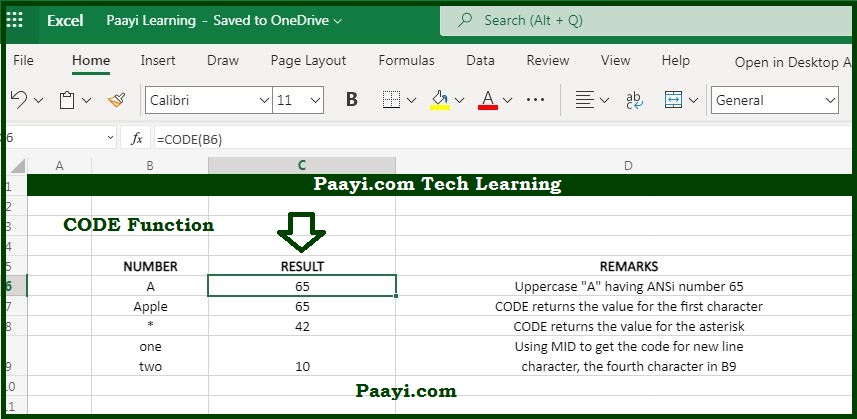# Learn How to Use Microsoft Excel CODE Function

Written by | 0 Comments | 726 Views

In this article, you will learn how to use the Microsoft Excel CODE function and its prime function in Microsoft Excel. You will also get to know the Microsoft Excel CODE function return value and syntax with the help of some examples.

## Microsoft Excel Code Function

The primary use of the CODE function is to get a code for a character. That means the Microsoft Excel CODE function returns the numeric code for a given character. You can also use the CODE function to find out the numeric codes supplied to the CODE function.

### CODE Function Return Value

It will return a numeric code corresponding to the character.

### CODE Syntax

= CODE (text)

Where the argument:

• text: The text for which you want to get a numeric code.

## How to Use Microsoft Excel CODE Function?Using the CODE function, you can able to return the numeric code for the first character in a string where the code is based on the character set used by your specific computer.